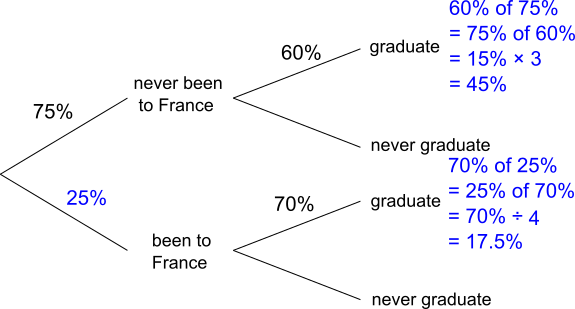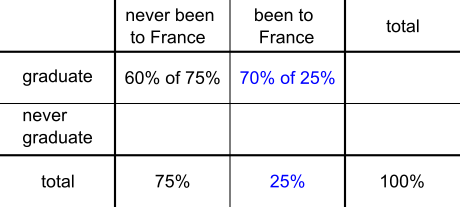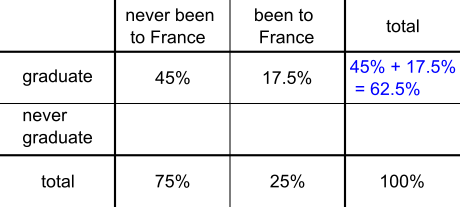#### You may also like### In a Box

Chris and Jo put two red and four blue ribbons in a box. They each pick a ribbon from the box without looking. Jo wins if the two ribbons are the same colour. Is the game fair?### Chances Are

Which of these games would you play to give yourself the best possible chance of winning a prize?### Which Spinners?

Can you work out which spinners were used to generate the frequency charts?

# Dropouts

##### Age 14 to 16 ShortChallenge Level

Using a tree diagram
The students at the language school can be represented using a tree diagram, as shown:The missing percentages can then be calculated:So a total of 45% + 17.5% = 62.5% of students graduate.

45% have never been to France and graduated, so we need to find 45% as a percentage of 62.5%: $$\frac{45}{62.5}=\frac{?}{100}\\ \Rightarrow \frac {90}{125}=\frac{?}{100}\\ \Rightarrow \frac {90\times100}{125}=?\\ \Rightarrow \frac{18\times5\times25\times4}{5\times25}=?\\ \Rightarrow 18\times4=?$$
So 72% of students who graduate have never been to France.

Using a two-way table
The students at the language school can be represented using a two-way table, as shown:60% of 75% can be found as half of 75% plus 10% of 75% = 37.5% + 7.5% = 45%,
or as 75% of 60% = 3 $\times$ 15% = 45%.

70% of 25% can be found as half of 25% plus a fifth of 25% = 12.5% + 5% = 17.5%,
or as 25% of 70% = 70% $\div$ 4 = 17.5%.

Then we can find the total percentage of students who graduate:A total 62.5% of students graduate, of whom 45% have never been to France, so we need to find 45% as a percentage of 62.5%: $$\frac{45}{62.5}=\frac{?}{100}\\ \Rightarrow \frac {90}{125}=\frac{?}{100}\\ \Rightarrow \frac {90\times100}{125}=?\\ \Rightarrow \frac{18\times5\times25\times4}{5\times25}=?\\ \Rightarrow 18\times4=?$$
So 72% of students who graduate have never been to France.

Using a Venn diagram
The students at the language school can be represented using a Venn diagram, as shown:The missing percentages can be calculated:So a total of 45% + 17.5% = 62.5% of students graduate.

45% have never been to school and graduated, so we need to find 45% as a percentage of 62.5%: $$\frac{45}{62.5}=\frac{?}{100}\\ \Rightarrow \frac {90}{125}=\frac{?}{100}\\ \Rightarrow \frac {90\times100}{125}=?\\ \Rightarrow \frac{18\times5\times25\times4}{5\times25}=?\\ \Rightarrow 18\times4=?$$
So 72% of students who graduate have never been to France.

You can find more short problems, arranged by curriculum topic, in our short problems collection.